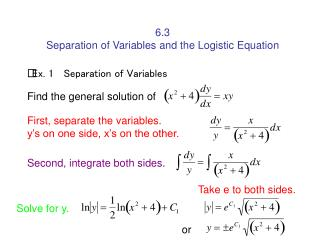DownloadDownload Presentation6.3 Separation of Variables and the Logistic Equation

# 6.3 Separation of Variables and the Logistic Equation

Télécharger la présentation## 6.3 Separation of Variables and the Logistic Equation

- - - - - - - - - - - - - - - - - - - - - - - - - - - E N D - - - - - - - - - - - - - - - - - - - - - - - - - - -
##### Presentation Transcript

1. 6.3 Separation of Variables and the Logistic Equation Ex. 1 Separation of Variables Find the general solution of First, separate the variables. y’s on one side, x’s on the other. Second, integrate both sides. Take e to both sides. Solve for y. or

2. Ex. 2 Finding a Particular Solution Given the initial condition y(0) = 1, find the particular solution of the equation To separate the variables, you must rid the first term of y and the second term of e-x^2. To do this, multiply both sides by ex^2/y. Now, integrate both sides.

3. u = x2 du = 2x dx du/2x = dx Now find C at (0,1) or by multiplying by 2, you get

4. Ex. 3 Finding a Particular Solution Curve Find the equation of the curve that passes through the point (1,3) and has a slope of y/x2 at any point (x,y). Because the slope is y/x2, you have Now, separate the variables. Take e to both sides to solve for y. at the point (1,3), C = ? or So, the equation is

5. Ex. 4. Wildlife Population The rate of change of the number of coyotes N(t) in a population is directly proportional to 650 - N(t), where t is the time in years. When t = 0, the population is 300, and when t = 2, the population has increased to 500. Find the population when t = 3. Because the rate of change of the population is proportional to 650 - N(t), we can write the following differential equation. Separate variables Integrate

6. Take e to both sides. Using N = 300 when t = 0, you can conclude that C = 350, which produces Then, using N = 500 when t = 2, it follows that So, the model for the coyote population is When t = 3, the approximate population is